# Chemical Reactions Chemical Reactions Chemical reactions Substances go

• Slides: 17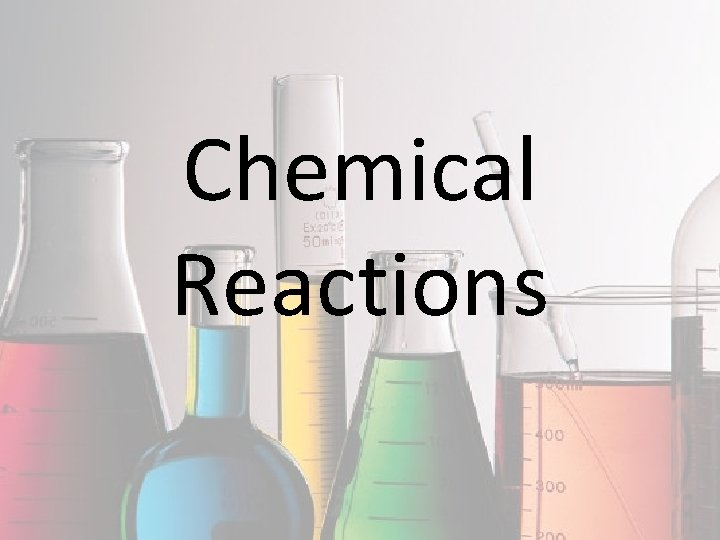Chemical Reactions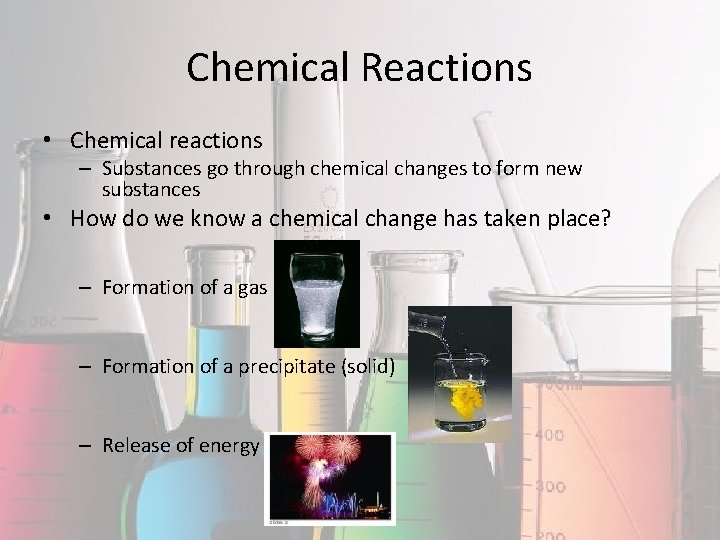Chemical Reactions • Chemical reactions – Substances go through chemical changes to form new substances • How do we know a chemical change has taken place? – Formation of a gas – Formation of a precipitate (solid) – Release of energy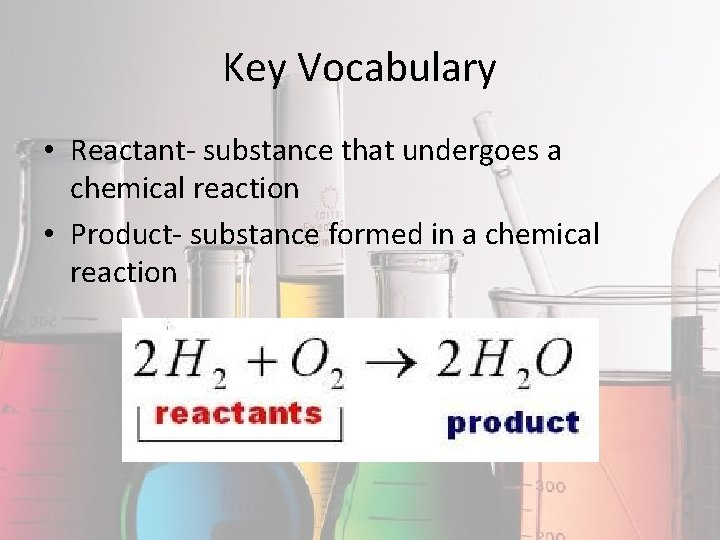Key Vocabulary • Reactant- substance that undergoes a chemical reaction • Product- substance formed in a chemical reaction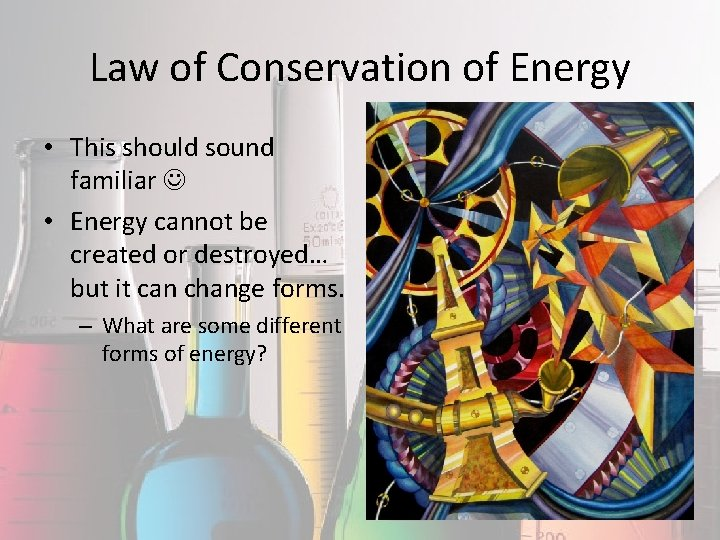Law of Conservation of Energy • This should sound familiar • Energy cannot be created or destroyed… but it can change forms. – What are some different forms of energy?Chemical Reactions • Two basic changes in energy: –Exothermic –EndothermicExothermic Reactions • Energy is released during the reaction – Heat, light, electricity, etc.Endothermic Reactions • Energy is absorbed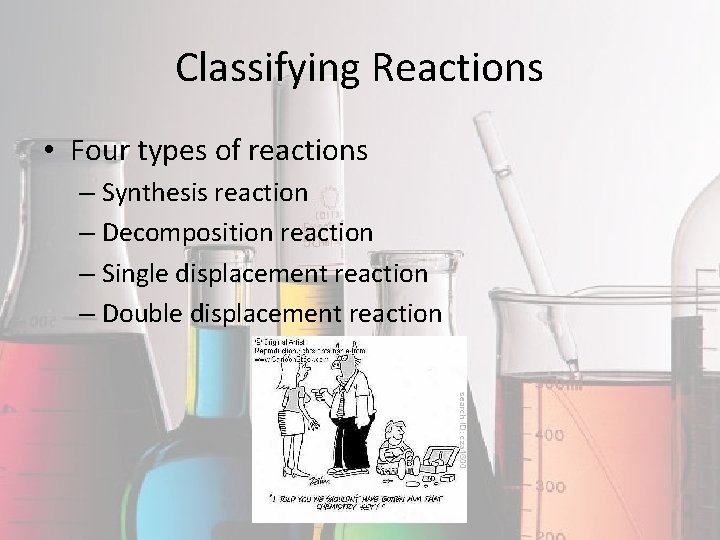Classifying Reactions • Four types of reactions – Synthesis reaction – Decomposition reaction – Single displacement reaction – Double displacement reactionSynthesis Reaction • Two or more substances combine to form a new compound • The product is more complex than the reactants • Formula generally formatted like this: A + B AB – Ex: 2 H + O H 2 O, Na + Cl Na. Cl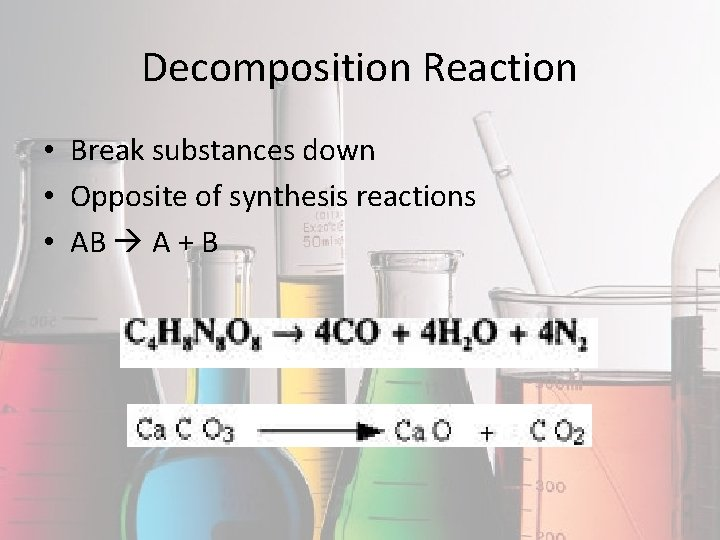Decomposition Reaction • Break substances down • Opposite of synthesis reactions • AB A + B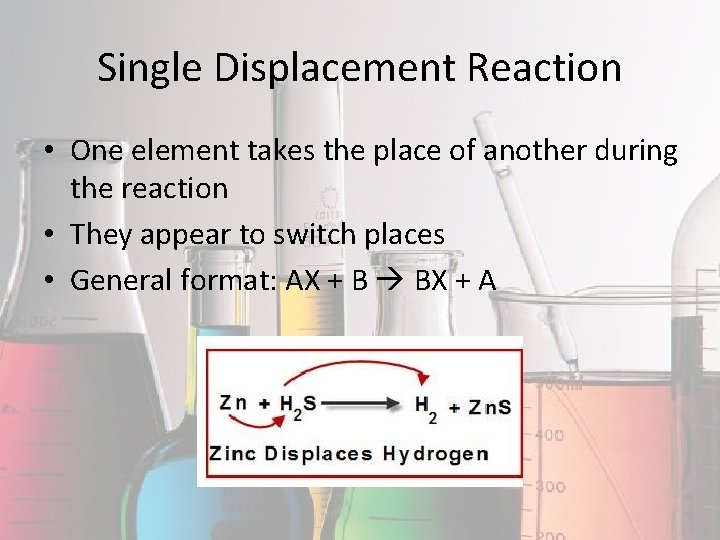Single Displacement Reaction • One element takes the place of another during the reaction • They appear to switch places • General format: AX + B BX + ADouble Displacement Reaction • Two compounds appear to exchange ions • General format: AX + BY AY + BX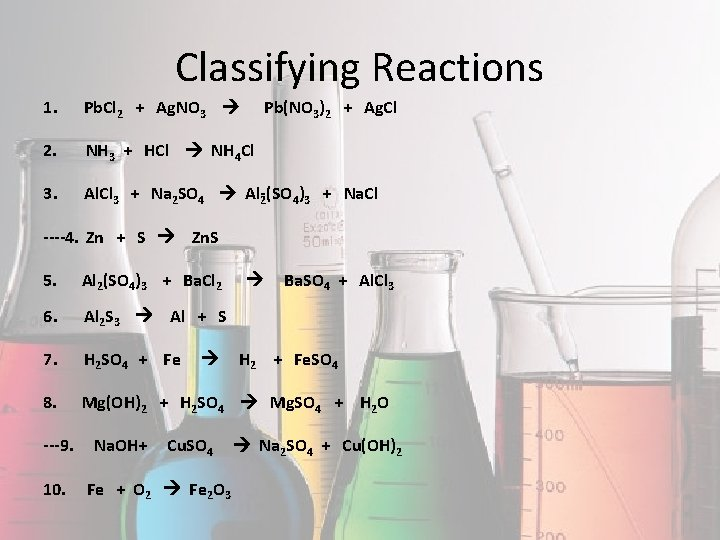Classifying Reactions 1. Pb. Cl 2 + Ag. NO 3 2. NH 3 + HCl NH 4 Cl 3. Al. Cl 3 + Na 2 SO 4 Al 2(SO 4)3 + Na. Cl Pb(NO 3)2 + Ag. Cl 4. Zn + S Zn. S 5. Al 2(SO 4)3 + Ba. Cl 2 6. Al 2 S 3 Al + S 7. H 2 SO 4 + Fe 8. Mg(OH)2 + H 2 SO 4 Mg. SO 4 + H 2 O 9. 10. Na. OH+ Cu. SO 4 Fe + O 2 Fe 2 O 3 Ba. SO 4 + Al. Cl 3 H 2 + Fe. SO 4 Na 2 SO 4 + Cu(OH)2Balancing Equations • Chemical equations are like recipes – They tell us how many atoms of each element are needed for the reaction. • The Law of Conservation of Mass tells us that mass cannot be created or destroyed. • That means that in a chemical equation, there needs to be the same amount of an element on each side (reactants and products). • The equation needs to be balanced.How do We Balance Equations? • Count the atoms of each element on each side of the equation. And create an “inventory” of atoms. • To balance the equation, we can only add Coefficients. • We cannot change the subscripts that are already there.Practice • Mg + O 2 Mg. O – How many magnesium atoms do we have? – How many oxygen atoms? – What coefficients can we add to make both sides equal? • Na + Mg. F 2 Na. F + Mg • N 2 + H 2 NH 3 • Zn + Ag. NO 3 Zn(NO 3)2 + Ag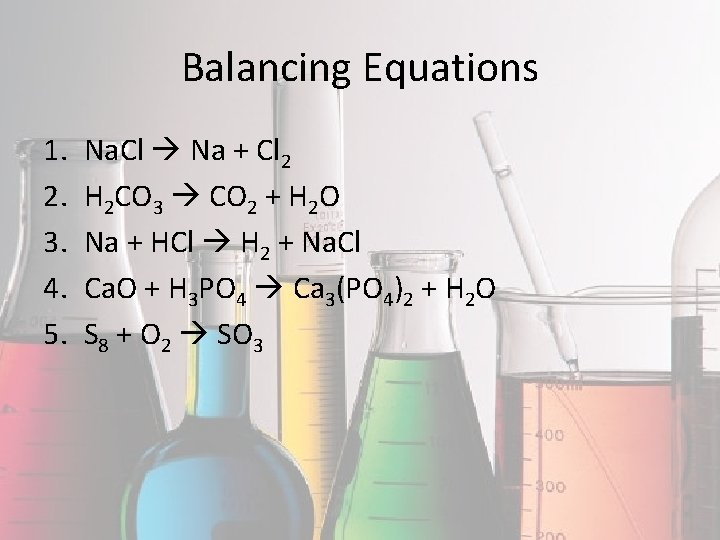Balancing Equations 1. 2. 3. 4. 5. Na. Cl Na + Cl 2 H 2 CO 3 CO 2 + H 2 O Na + HCl H 2 + Na. Cl Ca. O + H 3 PO 4 Ca 3(PO 4)2 + H 2 O S 8 + O 2 SO 3